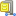### Abstract

We consider the problem of learning robust models of robot motion through demonstration. An approach based on Hidden Markov Model (HMM) and Gaussian Mixture Regression (GMR) is proposed to extract redundancies across multiple demonstrations, and build a time-independent model of a set of movements demonstrated by a human user. Two experiments are presented to validate the method, that consist of learning to hit a ball with a robotic arm, and of teaching a humanoid robot to manipulate a spoon to feed another humanoid. The experiments demonstrate that the proposed model can efficiently handle several aspects of learning by imitation. We first show that it can be utilized in an unsupervised learning manner, where the robot is autonomously organizing and encoding variants of motion from the multiple demonstrations. We then show that the approach allows to robustly generalize the observed skill by taking into account multiple constraints in task space during reproduction.

### Bibtex reference

@inproceedings{Calinon09Hum,
author = "Calinon, S. and D'halluin, F. and Caldwell, D.G. and Billard, A.",
title = "Handling of multiple constraints and motion alternatives in a robot programming by demonstration framework",
booktitle = "Proc. {IEEE-RAS} Intl Conf. on Humanoid Robots ({H}umanoids)",
year = "2009",
month="December",
location="Paris, France",
pages="582--588"
}

### Video

A HOAP-3 humanoid robot is used in the experiment. It has in total 28 DOFs, of which the 8 DOFs of the upper torso are used in the experiment. The experiment consists of feeding a Robota robotic doll, where HOAP-3 first brings a spoon to a plate of mashed potatoes and then moves it towards Robota's mouth.

This experiment aims at demonstrating that the framework can be used to learn a controller by taking into account several constraints. Here, we consider the case where a set of movements relative to a set of landmarks must be taken into consideration for the reproduction of the skill (i.e., where several actions on objects are considered).

### Source codesÂ  Download GMR Dynamics sourcecode

#### Usage

Unzip the file and run 'demo1' in Matlab.

#### Reference

• Calinon, S., D'halluin, F., Sauser, E.L., Caldwell, D.G. and Billard, A.G. (2009) A probabilistic approach based on dynamical systems to learn and reproduce gestures by imitation. IEEE Robotics and Automation Magazine.

#### Demo 1 - Demonstration of a trajectory learning system robust to perturbation based on Gaussian Mixture Regression (GMR)This program first encodes a trajectory represented through time 't', position 'x' and velocity 'dx' in a joint distribution P(t,x,dx) through Gaussian Mixture Model (GMM) by using Expectation-Maximization (EM) algorithm. Gaussian Mixture Regression (GMR) is then used to estimate P(x,dx|t), which retrieves another GMM refining the joint distribution model of position and velocity.
The learned skill can then be reproduced by combining an estimation of P(dx|x) with an attractor to the demonstrated trajectories.